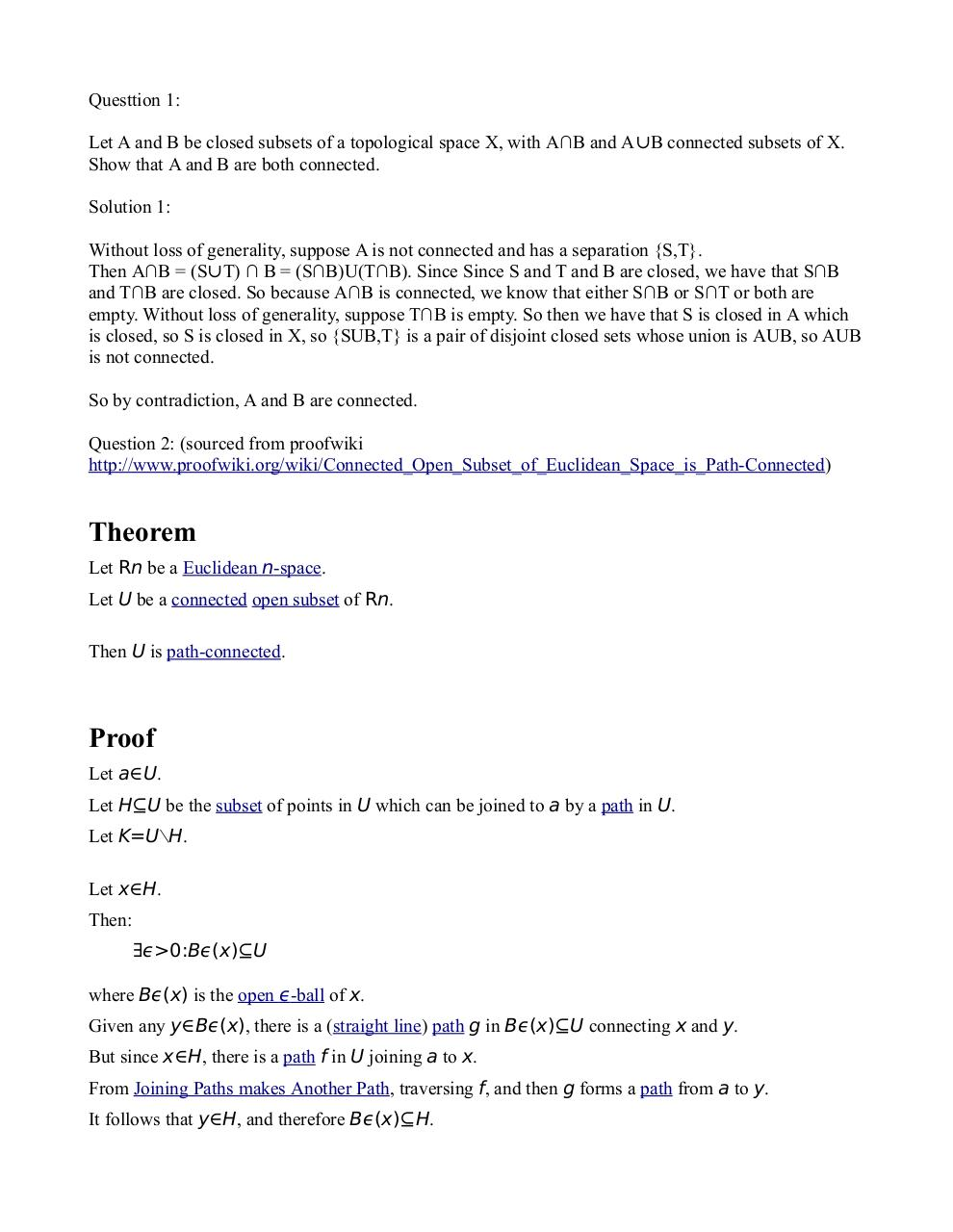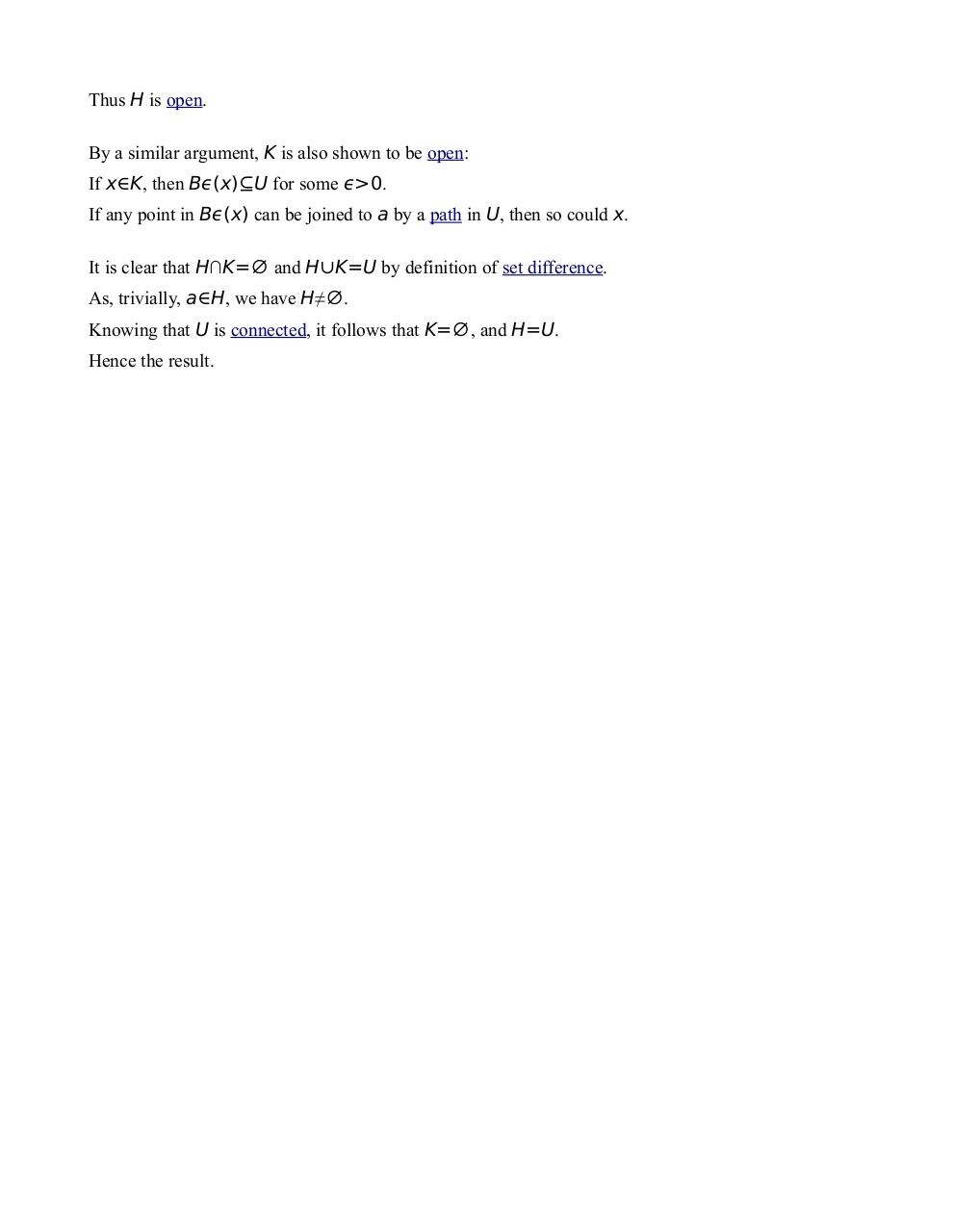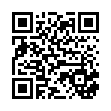# SubmittedQuestions .pdf

### File information

Original filename: SubmittedQuestions.pdf
Author: Josh

This PDF 1.4 document has been generated by Writer / LibreOffice 4.0, and has been sent on pdf-archive.com on 17/04/2014 at 19:07, from IP address 24.76.x.x. The current document download page has been viewed 456 times.
File size: 81 KB (2 pages).
Privacy: public file

SubmittedQuestions.pdf (PDF, 81 KB)

### Document preview

Questtion 1:
Let A and B be closed subsets of a topological space X, with A∩B and A∪B connected subsets of X.
Show that A and B are both connected.
Solution 1:
Without loss of generality, suppose A is not connected and has a separation {S,T}.
Then A∩B = (S∪T) ∩ B = (S∩B)U(T∩B). Since Since S and T and B are closed, we have that S∩B
and T∩B are closed. So because A∩B is connected, we know that either S∩B or S∩T or both are
empty. Without loss of generality, suppose T∩B is empty. So then we have that S is closed in A which
is closed, so S is closed in X, so {SUB,T} is a pair of disjoint closed sets whose union is AUB, so AUB
is not connected.
So by contradiction, A and B are connected.
http://www.proofwiki.org/wiki/Connected_Open_Subset_of_Euclidean_Space_is_Path-Connected)

Theorem
Let Rn be a Euclidean n-space.
Let U be a connected open subset of Rn.
Then U is path-connected.

Proof
Let a∈U.
Let H⊆U be the subset of points in U which can be joined to a by a path in U.
Let K=U∖H.
Let x∈H.
Then:
∃ϵ&gt;0:Bϵ(x)⊆U
where Bϵ(x) is the open ϵ-ball of x.
Given any y∈Bϵ(x), there is a (straight line) path g in Bϵ(x)⊆U connecting x and y.
But since x∈H, there is a path f in U joining a to x.
From Joining Paths makes Another Path, traversing f, and then g forms a path from a to y.
It follows that y∈H, and therefore Bϵ(x)⊆H.

Thus H is open.
By a similar argument, K is also shown to be open:
If x∈K, then Bϵ(x)⊆U for some ϵ&gt;0.
If any point in Bϵ(x) can be joined to a by a path in U, then so could x.
It is clear that H∩K=∅ and H∪K=U by definition of set difference.
As, trivially, a∈H, we have H≠∅.
Knowing that U is connected, it follows that K=∅, and H=U.
Hence the result.#### HTML Code

Copy the following HTML code to share your document on a Website or Blog

#### QR Code### Related keywords